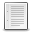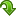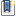Scilab Home Page | Wiki | Bug Tracker | Forge | Mailing List Archives | Scilab Online Help | ATOMS
File : Details
Please login or create an account

# polyroots()

Multiplicities and values of polynomial multiple roots
(883 downloads for this version - 883 downloads for all versions)
Details
Version
1.0
Author
Samuel Gougeon
Maintainer
Samuel Gougeon
Category
License
Supported Scilab Version
5.5
Creation Date
June 16, 2015Description
```            polyroots(..) identifies main simple or multiple roots of a polynomial, and
associated multiplicities.

-->uman polyroots

function [RootsMult] = polyroots(rp,rounded?)

CALLING SEQUENCE
polyroots   // displays this help
RootsMult = polyroots(rp, rounded?)

PARAMETERS
rp       : Polynomial (degree N) that's roots are searched for. Or,
Vector or matrix of N complex roots of a polynomial
of degree N (as coming from roots()).
rounded? : Scalar boolean. if True, main roots (in RootsMult(:,1))
are rounded to the %eps^(1/N)/10 relative accuracy.
RootsMult: Nx2 matrix of real or complex numbers.
Column #1: Average gathered roots ("main roots")
Column #2: Related multiplicities

DESCRIPTION
When numerically computing the N roots of a polynomial of degree N
with a floating point accuracy of %eps (epsilon-machine), the
relative accuracy of each computed root r can't be better than
%eps^(1/N), that is to say: the true root can be anywhere in a
disk centered on r and of radius |r|*%eps^(1/N).
Hence, for a root r of multiplicity m, the m computed raw roots
coming out of roots() will lay in a disk centered on r and of
radius |r|*%eps^(1/N).
After eventually calling roots(rp) whether rp is a polynomial,
polyroots() looks for clusters of raw roots within the |r|*%eps^(1/N)
vicinity criterion. Then, for each identified cluster, the actual
main root is computed as the average of raw roots covered by the disk,
and the number of raw roots covered sets main root's multiplicity.

NOTE: When true roots become too near from each others, they
might turn numerically undistinguishable. Returned main roots
may then merge several true ones with cumulated multiplicities.
All from roots() as well as from polyroots() are numerically correct.

EXAMPLES
// Example #1:  roots = 0, (0.5, x14), (%pi, x15)
p = (%s - %pi)^15 * (%s - 0.5)^14 * %s;
r = roots(p)       // raw roots from roots()
RM = polyroots(p)  // main roots from polyroots()

// Example #2:  roots = (%i, x7), (-%i, x6)
p = (%z -%i)^7 * (%z+%i)^6;
r = roots(p)       // raw roots from roots()
RM = polyroots(p)  // from polyroots()

// Example #3:  randomized roots
R = unique(grand(1,8,"uin",1,9));
M = grand(R,"uin",1,4);
gsort([R' M'],"lr")  // true roots and multiplicities
p = prod((%s - R).^M);
r = roots(p)       // raw roots from roots()
RM = polyroots(p)  // main roots from polyroots()

// Example #4:  root = (1-%i, x6), (2-3*%i, x4)
p = %s * (%s - 1+%i)^6 * (%s - 2+3*%i)^4;
r = roots(p)       // raw roots from roots()
RM = polyroots(p)  // main roots from polyroots()

// Example #5:  root = (0.789, x5),  (1.23456, x6)
p = (%s - 0.789)^5 * (%s - 1.23456)^6;
r = roots(p)       // raw roots from roots()
RM = polyroots(p)  // main roots from polyroots()

RESULTS
=======
// Example #1:  ROOTS = 0, (0.5, x14), (%pi, x15)
// ----------------------------------------------
--> p = (%s - %pi)^15 * (%s - 0.5)^14 * %s;
--> r = roots(p)       // raw roots from roots()
r  =
3.8711257
3.7851404 + 0.3295425i
3.7851404 - 0.3295425i
3.5568129 + 0.5695029i
3.5568129 - 0.5695029i
3.2575737 + 0.6681311i
3.2575737 - 0.6681311i
2.9654125 + 0.6215611i
2.9654125 - 0.6215611i
2.7422318 + 0.4599749i
2.7422318 - 0.4599749i
2.7927616
2.6243717 + 0.2368523i
2.6243717 - 0.2368523i
2.596916
0.6091467 + 0.0222994i
0.6091467 - 0.0222994i
0.5870115 + 0.0779843i
0.5870115 - 0.0779843i
0.5340530 + 0.1058458i
0.5340530 - 0.1058458i
0.4827582 + 0.1013348i
0.4827582 - 0.1013348i
0.4171420 + 0.0145190i
0.4171420 - 0.0145190i
0.4243227 + 0.0464079i
0.4243227 - 0.0464079i
0.4455662 + 0.0779780i
0.4455662 - 0.0779780i
0

--> RM = polyroots(p)  // main roots from polyroots()
RM  =
3.1415926    15.
0.5000000    14.
0            1.

// Example #2:  ROOTS = (%i, x7), (-%i, x6)
//-----------------------------------------
--> p = (%z -%i)^7 * (%z+%i)^6;
--> r = roots(p)       // raw roots from roots()
r  =
0.0016859 - 0.9990590i
0.0016540 - 1.0009888i
0.0000242 - 0.9980686i
- 0.0000266 - 1.0019238i
- 0.0016594 - 0.9990171i
- 0.0016782 - 1.0009427i
- 0.0055694 + 1.0011948i
- 0.0025364 + 1.0051147i
- 0.0043981 + 0.9964037i
0.0024321 + 1.0051666i
0.0000549 + 0.9943245i
0.0044695 + 0.9964889i
0.0055473 + 1.0013067i

--> RM = polyroots(p)  // from polyroots()
RM  =
i      7.
- i      6.

// Example #4:  ROOTS = (1-%i, x6), (2-3*%i, x4)
// --------------------------------------------
--> p = %s * (%s - 1+%i)^6 * (%s - 2+3*%i)^4;
--> r = roots(p)       // raw roots from roots()
r  =
1.9986192 - 2.9986152i
1.9986165 - 3.0013802i
2.0013855 - 2.9986205i
2.0013788 - 3.0013842i
0.9985528 - 1.0099018i
0.9907701 - 1.0036693i
0.9921979 - 0.9939138i
1.007921 - 1.0061746i
1.0013112 - 0.9901569i
1.0092471 - 0.9961836i
0

--> RM = polyroots(p)  // main roots from polyroots()
RM  =
2. - 3.i    4.
1. - i      6.
0           1.

// Example #5:  ROOTS = (0.789, x5),  (1.23456, x6)
// -----------------------------------------------
--> p = (%s - 0.789)^5 * (%s - 1.23456)^6;
--> r = roots(p)       // raw roots from roots()
r  =
1.2531698
1.2442363 + 0.0160970i
1.2442363 - 0.0160970i
1.2252945 + 0.0168015i
1.2252945 - 0.0168015i
1.2151286
0.7956003
0.7908533 + 0.0062082i
0.7908533 - 0.0062082i
0.7838467 + 0.0036353i
0.7838467 - 0.0036353i

--> RM = polyroots(p)  // main roots from polyroots()
RM  =
1.23456      6.
0.7890000    5.
```Files (1)Miscellaneous file
```Macro defining polyroots()

* MAKE IT AVAILABLE in EVERY SESSION: Click there to see indications

* Get help: Enter "polyroots" without parameters

```News (0)Comments (0)Leave a comment
You must register and log in before leaving a comment.Email notifications
Send me email when this toolbox has changes, new files or a new release.
You must register and log in before setting up notifications.